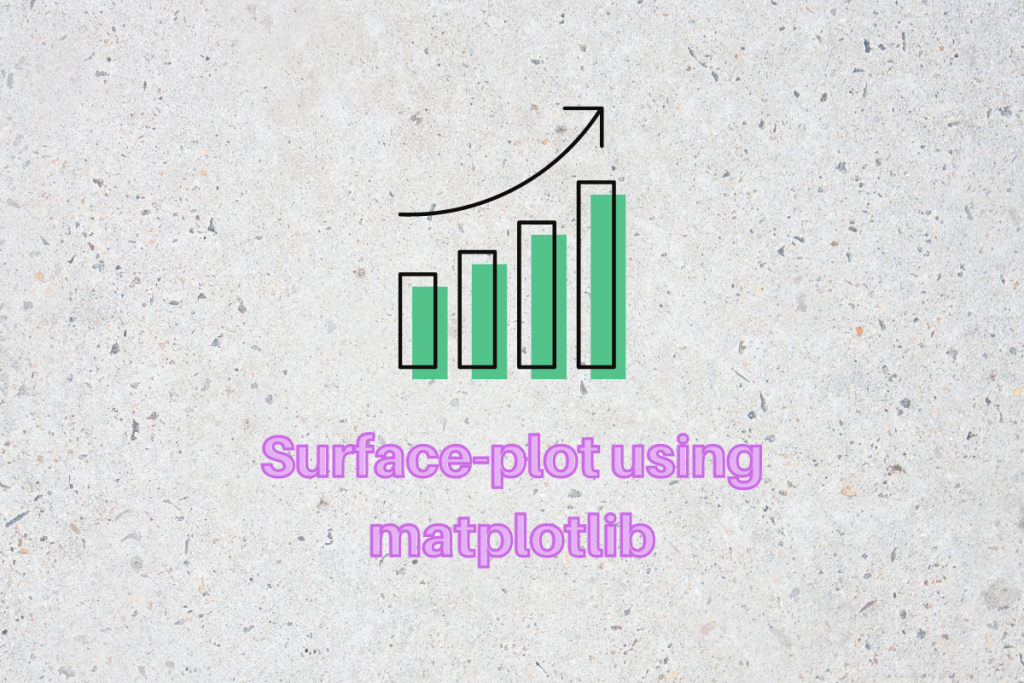# How to draw a surface plot in matplotlibWe have previously covered how to draw contour plots in matplotlib. Now it is time to learn about surface plots in matplotlib. Surface plots are a great way to visualize 3-dimensional data in a visually pleasing format. The different colour coding allows one to understand the variability of the data at different points. In mathematics, we use this very extensively.

## Drawing a surface plot in matplotlib using dummy data

First, let us draw a surface plot of some dummy data. We first import all the necessary libraries:

```import pandas as pd
import numpy as np
import matplotlib.pyplot as plt
```

Now let us create some dummy data:

```x = [1,2,3,4]
y = [5,7,10,12]
```

Surface plots require mesh data and we, therefore, need to convert the data into the correct format, for this we will use the np.meshgrid function:

```X, Y = np.meshgrid(x,y)
```

which gives us the following result:

```[array([[1, 2, 3, 4],
[1, 2, 3, 4],
[1, 2, 3, 4],
[1, 2, 3, 4]]),
array([[ 5,  5,  5,  5],
[ 7,  7,  7,  7],
[10, 10, 10, 10],
[12, 12, 12, 12]])]
```

We will now need to create a third variable Z since surface plots are done for 3-dimensional data sets. We will be using the X and Y variable to transform them into our Z variable. There are several functions that can be made, but we will go with a commonly used z-function, the code is as follows:

```def z_function(x, y):
return np.sin(np.sqrt(x**2+y**2))
Z = z_function(X, Y)
```

Now we have all three variables and we can draw our surface plot. To do that we execute the following code:

```plt.figure(figsize=(10, 10))
ax = plt.axes(projection='3d')
ax.plot_surface(X, Y, Z)
```

If we execute the above code the following figure is generated:

If you are using the Jupyter Notebook then you can add in the following command at the beginning of the last block and then you can even interact with the figure:

```matplotlib notebook
```

## Surface Plot using your own dataset (csv file)

Now, we won’t always know the function we need to plot. In fact, most of the time we will have some sample data at our disposal and be required to create a surface plot using that. To do that let us see the instructions.

First, we need to import the csv file into our python instance. To do this we will use the pd.read_csv function:

```df = pd.read_csv("name_of_csv.csv")
```

We can look at all the columns in the dataset by executing the following code:

```df.columns
```

We can now set the x and the y variables and again create the meshgrid:

```x = df['column_name_of_x']
y = df['column_name_of_y']
X, Y = np.meshgrid(x,y)
```

We will be required to again define our z function:

```Z = z_function(X, Y)
```

Now the rest is as before again, we initialize our axes and plot the data:

```plt.figure(figsize=(10, 10))
ax = plt.axes(projection='3d')
ax.plot_surface(X, Y, Z)
```

As a final touch let’s add in some labels and we will be done for the day!

```ax.set_xlabel("x-label")
ax.set_ylabel("y-label")
```

## Conclusion

So there you go, readers! You now have a basic understanding of how to draw a surface plot using matplotlib! If you’re looking forward to more such awesome content keep following AskPython, and as always, thanks for reading!